Categories

# Temperature, Pressure, and Density: The Equation of State

The state of the atmosphere is described by pressure p [Pa], temperature T [K], and chemical composition. Atmospheric pressure is sufficiently low that the ideal gas law is obeyed within 1% under all conditions. The equation of state can therefore be expressed as

pV = RT

where V [m3 mol–1] represents the molar volume of air, and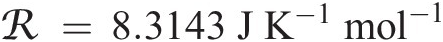is the universal gas constant. When expressed as a function of the number density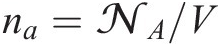[molecules m–3], where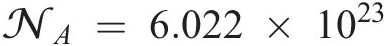molecules mol–1 is Avogadro’s number, this expression becomes

p = nakT

where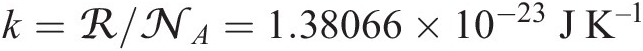is Boltzmann’s constant.The equation of state can also be expressed as a function of the mass density of air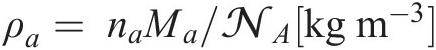p = ρaRT

where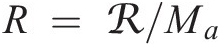is the specific gas constant for air and Ma [kg mol–1] is the molar mass of air.The molar mass of air is the weighted average of the mass of its components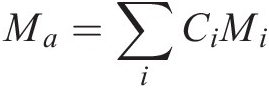where Ci and Mi are respectively the mole fraction (commonly called molar or volume mixing ratio) and the molar mass of constituent i. Since dry air can be closely approximated as a mixture of nitrogen N2 (with CN2 = 0.78), oxygen O2 (with CO2 = 0.21) and argon Ar (with CAr = 0.01), the molar mass for dry air is Md = 28.97 × 10–3 kg mol–1. Water vapor, which can account for up to a few percent of air in the lower troposphere, will make air slightly lighter.